Stoichiometry What is stoichiometry stoichiometry method of determining

• Slides: 14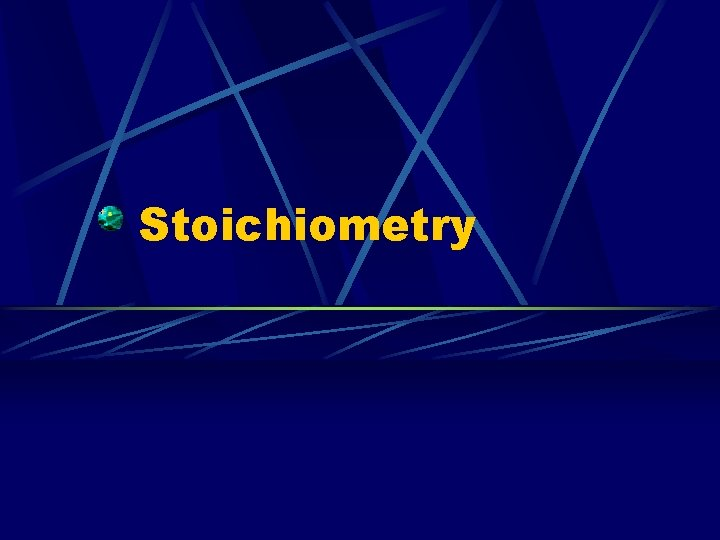Stoichiometry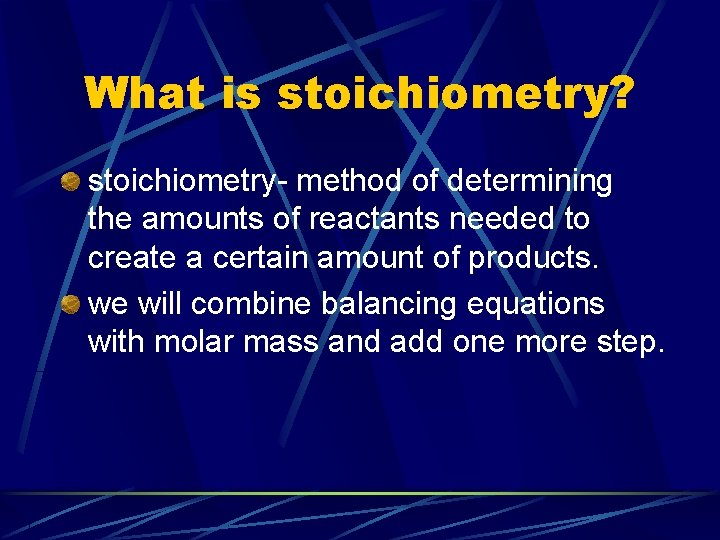What is stoichiometry? stoichiometry- method of determining the amounts of reactants needed to create a certain amount of products. we will combine balancing equations with molar mass and add one more step.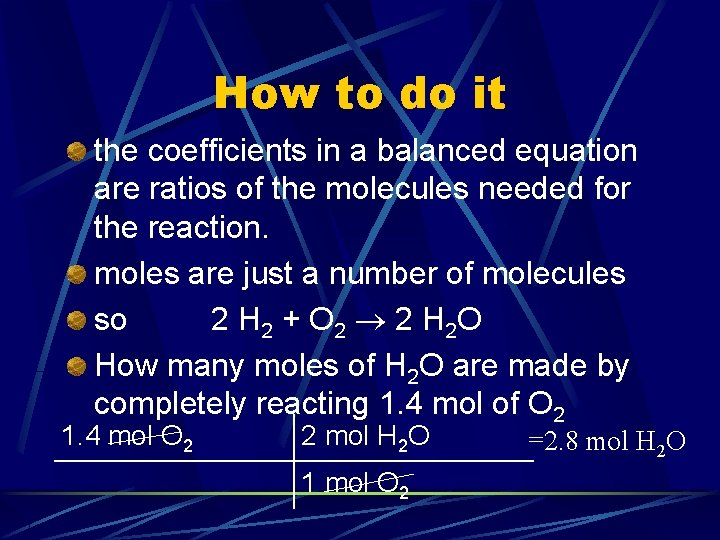How to do it the coefficients in a balanced equation are ratios of the molecules needed for the reaction. moles are just a number of molecules so 2 H 2 + O 2 2 H 2 O How many moles of H 2 O are made by completely reacting 1. 4 mol of O 2 1. 4 mol O 2 2 mol H 2 O 1 mol O 2 =2. 8 mol H 2 OAdding in molar mass How many grams of H 2 are needed to completely react with 24 g of O 2? 2 H 2 + O 2 2 H 2 O convert to moles convert to the desired molecule convert to grams O 2 = 32. 00 g/mol ; H 2 = 2. 016 g/mol 24 g O 2 1 mol O 2 2 mol H 2 32 g O 2 1 mol O 2 2. 016 g H 2 1 mol H 2 = 3. 0 g H 2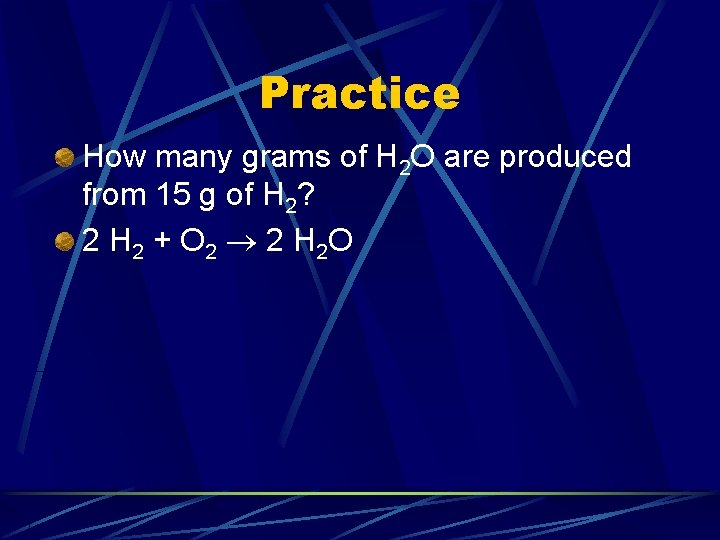Practice How many grams of H 2 O are produced from 15 g of H 2? 2 H 2 + O 2 2 H 2 O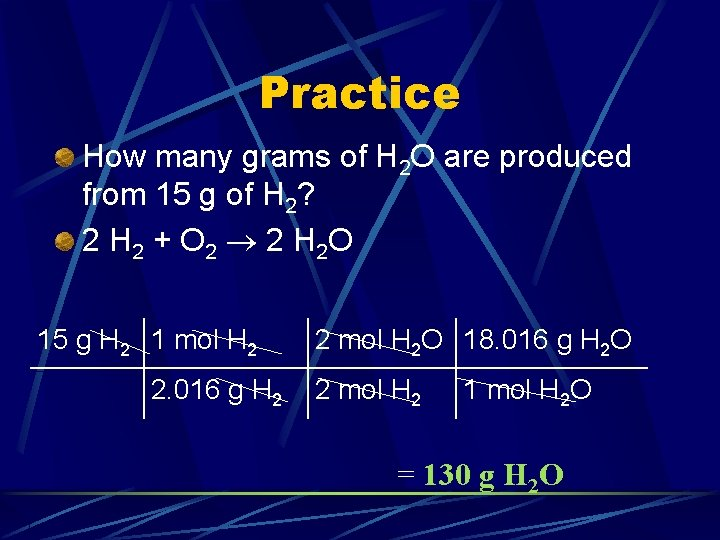Practice How many grams of H 2 O are produced from 15 g of H 2? 2 H 2 + O 2 2 H 2 O 15 g H 2 1 mol H 2 2. 016 g H 2 2 mol H 2 O 18. 016 g H 2 O 2 mol H 2 1 mol H 2 O = 130 g H 2 Opractice How many grams of H 2 O are produced by completely reacting 24 g of O 2? 2 H 2 + O 2 2 H 2 O convert to moles convert to the desired molecule convert to grams O 2 = 32. 00 g/mol ; H 2 O = 18. 016 g/molpractice How many grams of H 2 O are produced by completely reacting 24 g of O 2? 2 H 2 + O 2 2 H 2 O convert to moles convert to the desired molecule convert to grams O 2 = 32. 00 g/mol ; H 2 O = 18. 016 g/mol 24 g O 2 1 mol O 2 2 mol H 2 O 18. 016 g H 2 O = 27 g H 2 O 32 g O 1 mol H O 2 2 2Another How many grams of H 2 are needed to form 34 g of H 2 O? 2 H 2 + O 2 2 H 2 O convert to moles convert to the desired molecule convert to grams H 2 = 2. 016 g/mol ; H 2 O = 18. 016 g/molAnother How many grams of H 2 are needed to form 34 g of H 2 O? 2 H 2 + O 2 2 H 2 O convert to moles convert to the desired molecule convert to grams H 2 = 2. 016 g/mol ; H 2 O = 18. 016 g/mol 34 g H 2 O 1 mol H 2 O 2 mol H 2 2. 016 g H 2 = 3. 8 g 18. 016 g H 2 O 2 mol H 2 O 1 mol H 2Balance the equation 1 st, then do the problem Na + Cl 2 Na. Cl How many grams of chlorine are needed to react with 37 g of Na?Balance the equation 1 st, then do the problem 2 Na + Cl 2 2 Na. Cl How many grams of chlorine are needed to react with 37 g of Na? 37 g Na 1 mol Cl 2 22. 99 g Na 2 mol Na =57 g Cl 2 70. 90 g Cl 2 1 mol Cl 2More CH 4 + O 2 H 2 O + CO 2 How many grams of CH 4 are needed to make 120 g of H 2 O?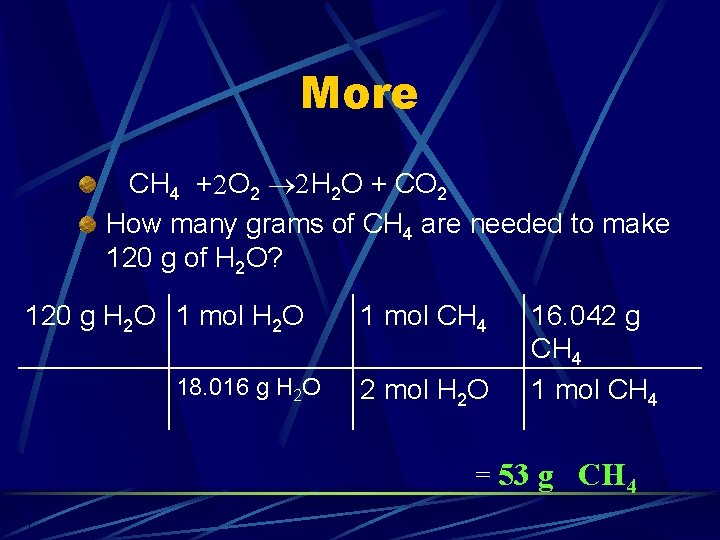More CH 4 +2 O 2 2 H 2 O + CO 2 How many grams of CH 4 are needed to make 120 g of H 2 O? 120 g H 2 O 1 mol H 2 O 18. 016 g H 2 O 1 mol CH 4 2 mol H 2 O 16. 042 g CH 4 1 mol CH 4 = 53 g CH 4